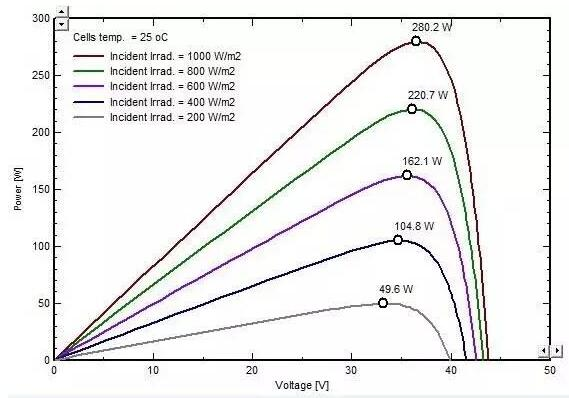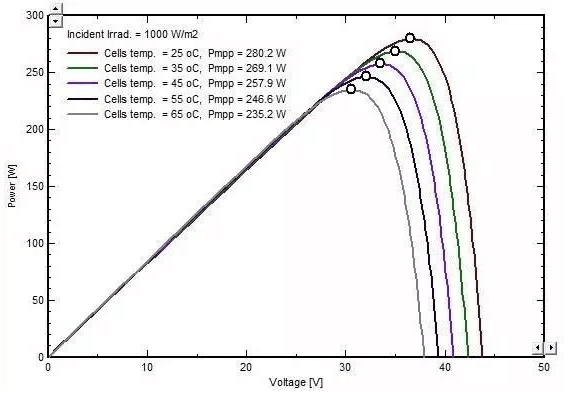You can share

• Share to Facebook
• Share to Google+
• Subscribe to our
• Share to Linkedin
• Share to Twitter
30000 hours of trouble-free operation inverter manufacturersMPPT is a very core technology of inverter. MPPT voltage is a key parameter in the design of photovoltaic power station.

# Function, principle and algorithm of pv inverter MPPT

• Xindun
• September 26, 2021

MPPT is a very core technology of inverter. MPPT voltage is a key parameter in the design of photovoltaic power station.

(The i-v and p-v curves of a single photovoltaic module)

The figure above, photovoltaic modules of output voltage and current to follow the i-v curve (green), P - V curves (blue), if you want the inverter output power is the largest, requires dc voltage running in the red dot was one of the biggest point, the point is the point of maximum power. If the maximum power point is 550V, the power at 550V is 200W. At this point, the power running at 520V is about 190W, while that running at 580V is about 185W, neither of which is as high as 550V. If the inverter tracks less than 550V, it loses power, but it has no other impact on the system.

So why keep tracking? Because the curve as the light intensity, temperature, and different in the changing of shade, also in change of maximum power point, possible maximum power point voltage is 560 v in the morning, noon is 520 v, is 550 v in the afternoon, so the inverter need to constantly looking for the maximum power point, also is the maximum power point tracking, so as to ensure the panels of energy throughout the day can maximize the output, and not waste of solar energy resources.

After the above basic knowledge, let\'s talk about MPPT.

Short for MPPT, namely MaximumPowerPointTracking, it refers to the inverter according to the different environment, such as temperature, light intensity of the outside world features to adjust the output power of pv array, make pv array maximum power output.

Due to the received light intensity of solar battery and environmental impact of external factors, such as the output of changes, the light intensity of electricity is much, the most power tracing of the inverter with MPPT is in order to make full use of solar cells, and make it run at maximum power point. In other words, if the solar radiation is constant, the output power after MPPT will be higher than that before MPPT, which is the function of MPPT.

For example, suppose that MPPT hasn\'t started tracking, when the component output voltage is 500 v, then the MPPT began tracking, started by the internal circuit structure adjust of resistance on the back, to change the component output voltage, change the output current at the same time, all the way to the output power (assuming is 550 v), the largest since then have been tracking, so that is under the condition of invariable in solar radiation, components in the output voltage is 550 v, output power will be higher than 500 v, this is the function of MPPT.

Factors influencing MPPT of a single photovoltaic module

Generally speaking, the influence of irradiance and temperature change on output power is most directly reflected in MPPT, that is to say, irradiance and temperature are important factors influencing MPPT.

The output power of photovoltaic modules will be reduced when the irradiance decreases. As the temperature rises, the output power of the photovoltaic module will decrease.

1. Effect of irradiation on MPPT(Effect of irradiance change on the output power of photovoltaic modules)

The maximum power point tracking (MPPT) of the inverter is to find the maximum power point in the figure above. As can be seen from the figure above, when the irradiance decreases, the maximum power point is reduced almost proportionally, as shown in the following table.

 Irradiance（W/m²） Maximum power（W） Output power ratio 1000 280.2 1 800 220.7 0.79 600 162.1 0.58 400 104.8 0.37 200 49.6 0.18

As can be seen from the above table, when the irradiance decreases in proportion, the MPPT voltage drops slightly. Since P=IU, when the irradiance decreases, P decreases proportionately, while U decreases slightly, so current-I should decrease proportionately.

2. The influence of temperature on MPPT(Influence of temperature change on output power of components)

As can be seen from the figure above, when the temperature changes, the maximum power decreases gradually in proportion to the temperature coefficient, as shown in the following table.

 Temperature（℃） Maximum power（W） The number of degrees of increase relative to 25 Power reduction ratio Power loss per liter of one degree Celsius 25 280.2 0 0 0 35 269.1 10 -4.0% -0.40% 45 257.9 20 -8.0% -0.40% 55 246.6 30 -12.0% -0.40% 65 235.2 40 -16.1% -0.40%

It can be seen that the current is mainly affected by the irradiance, and the voltage is mainly affected by the temperature.